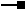# IXMLSerializeData3 Interface

## Members

Name DescriptionAddBinary Adds element value as an array of bytes.AddBoolean Adds element value as a boolean.AddByte Adds element value as a byte.AddDate Adds element value as a date.AddDouble Adds element value as a double.AddFloat Adds element value as a float.AddInt64 Adds element value as an int64.AddInteger Adds element value as an integer.AddObject Adds element value as an object.AddShort Adds element value as a short.AddString Adds element value as a string.AddUInteger Adds element value as an unsigned integer.AddUShort Adds element value as a unsigned short.AddVariant Adds element value as a variant.Count Number of XML elements.Find Finds an XML element by name.GetBinary Obtains element value as an array of bytes.GetBoolean Obtains element value as a boolean.GetByte Obtains element value as a byte.GetDate Obtains element value as a date.GetDouble Obtains element value as a double.GetFlag Obtains the value for a serialization flag.GetFloat Obtains element value as a float.GetInt64 Obtains element value as an int64.GetInteger Obtains element value as an integer.GetObject Obtains element value as an object instance.GetShort Obtains element value as a short.GetString Obtains element value as a string.GetUInteger Obtains element value as an unsigned integer.GetUShort Obtains element value as an unsigned short.GetVariant Obtains element value as a variant.Properties Properties for serialization and deserialization.SetFlag Writes the value for a serialization flag.TypeName XML type of the object.TypeName XML type of the object.TypeNamespaceURI XML type namespace of the object.TypeNamespaceURI XML type namespace of the object.

Adds element value as an unsigned integer.

``````Public Sub AddUInteger ( _
ByVal Name As String, _
ByVal Value As Integer _
)
``````
``````public void AddUInteger (
string Name,
uint Value
);
``````

Adds element value as a unsigned short.

``````Public Sub AddUShort ( _
ByVal Name As String, _
ByVal Value As ushort _
)
``````
``````public void AddUShort (
string Name,
ushort Value
);
``````

### IXMLSerializeData3.GetUInteger Method

Obtains element value as an unsigned integer.

``````Public Function GetUInteger ( _
ByVal Index As Integer _
) As Integer
``````
``````public uint GetUInteger (
int Index
);
``````

### IXMLSerializeData3.GetUShort Method

Obtains element value as an unsigned short.

``````Public Function GetUShort ( _
ByVal Index As Integer _
) As ushort
``````
``````public ushort GetUShort (
int Index
);
``````

## Inherited Interfaces

Interfaces Description
IXMLSerializeData2 Provides access to members that serialize and deserialize data from XML.
IXMLSerializeData Provides access to members that serialize and deserialize data from XML.

## Classes that implement IXMLSerializeData3

Classes Description